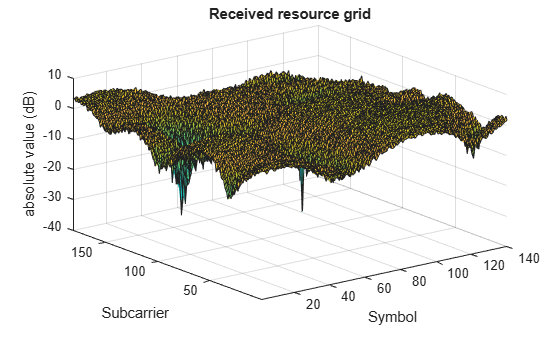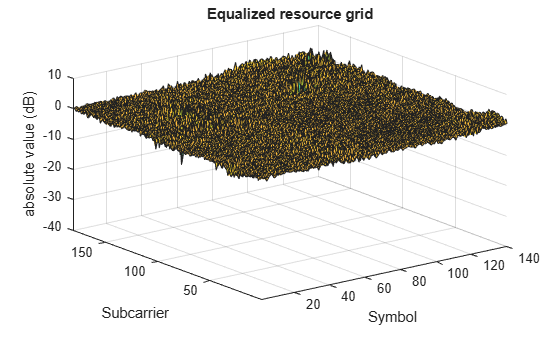# LTE Downlink Channel Estimation and Equalization

This example shows how to use the LTE Toolbox™ to create a frame worth of data, pass it through a fading channel and perform channel estimation and equalization. Two figures are created illustrating the received and equalized frame.

### Introduction

This example shows how a simple transmitter-channel-receiver simulation may be created using functions from the LTE Toolbox. The example generates a frame worth of data on one antenna port. As no transport channel is created in this example the data is random bits, QPSK modulated and mapped to every symbol in a subframe. A cell specific reference signal and primary and secondary synchronization signals are created and mapped to the subframe. 10 subframes are individually generated to create a frame. The frame is OFDM modulated, passed through an Extended Vehicular A Model (EVA5) fading channel, additive white Gaussian noise added and demodulated. MMSE equalization using channel and noise estimation is applied and finally the received and equalized resource grids are plotted.

### Cell-Wide Settings

The cell-wide settings are specified in a structure `enb`. A number of the functions used in this example require a subset of the settings specified below. In this example only one transmit antenna is used.

```enb.NDLRB = 15; % Number of resource blocks enb.CellRefP = 1; % One transmit antenna port enb.NCellID = 10; % Cell ID enb.CyclicPrefix = 'Normal'; % Normal cyclic prefix enb.DuplexMode = 'FDD'; % FDD```

### SNR Configuration

The operating SNR is configured in decibels by the value `SNRdB` which is also converted into a linear SNR.

```SNRdB = 22; % Desired SNR in dB SNR = 10^(SNRdB/20); % Linear SNR rng('default'); % Configure random number generators```

### Channel Model Configuration

The channel model is configured using a structure. In this example a fading channel with an Extended Vehicular A (EVA) delay profile and 120Hz Doppler frequency is used. These parameters along with MIMO correlation and other channel model specific parameters are set.

```cfg.Seed = 1; % Channel seed cfg.NRxAnts = 1; % 1 receive antenna cfg.DelayProfile = 'EVA'; % EVA delay spread cfg.DopplerFreq = 120; % 120Hz Doppler frequency cfg.MIMOCorrelation = 'Low'; % Low (no) MIMO correlation cfg.InitTime = 0; % Initialize at time zero cfg.NTerms = 16; % Oscillators used in fading model cfg.ModelType = 'GMEDS'; % Rayleigh fading model type cfg.InitPhase = 'Random'; % Random initial phases cfg.NormalizePathGains = 'On'; % Normalize delay profile power cfg.NormalizeTxAnts = 'On'; % Normalize for transmit antennas```

### Channel Estimator Configuration

A user defined window is used to average pilot symbols to reduce the effect of noise. The averaging window size is configured in terms of resource elements (REs), in time and frequency. A conservative 9-by-9 window is used in this example as an EVA delay profile and 120Hz Doppler frequency cause the channel changes quickly over time and frequency. A 9-by-9 window includes the 4 pilots immediately surrounding the pilot of interest when averaging. Selecting an averaging window is discussed in Channel Estimation.

```cec.PilotAverage = 'UserDefined'; % Pilot averaging method cec.FreqWindow = 9; % Frequency averaging window in REs cec.TimeWindow = 9; % Time averaging window in REs```

Interpolation is performed by the channel estimator between pilot estimates to create a channel estimate for all REs. To improve the estimate multiple subframes can be used when interpolating. An interpolation window of 3 subframes with a centered interpolation window uses pilot estimates from 3 consecutive subframes to estimate the center subframe.

```cec.InterpType = 'Cubic'; % Cubic interpolation cec.InterpWinSize = 3; % Interpolate up to 3 subframes % simultaneously cec.InterpWindow = 'Centred'; % Interpolation windowing method```

### Subframe Resource Grid Size

In this example it is useful to have access to the subframe resource grid dimensions. These are determined using `lteDLResourceGridSize`. This function returns an array containing the number of subcarriers, number of OFDM symbols and number of transmit antenna ports in that order.

```gridsize = lteDLResourceGridSize(enb); K = gridsize(1); % Number of subcarriers L = gridsize(2); % Number of OFDM symbols in one subframe P = gridsize(3); % Number of transmit antenna ports```

### Transmit Resource Grid

An empty resource grid `txGrid` is created which will be populated with subframes.

`txGrid = [];`

As no transport channel is used in this example the data sent over the channel will be random QPSK modulated symbols. A subframe worth of symbols is created so a symbol can be mapped to every resource element. Other signals required for transmission and reception will overwrite these symbols in the resource grid.

```% Number of bits needed is size of resource grid (K*L*P) * number of bits % per symbol (2 for QPSK) numberOfBits = K*L*P*2; % Create random bit stream inputBits = randi([0 1], numberOfBits, 1); % Modulate input bits inputSym = lteSymbolModulate(inputBits,'QPSK');```

### Frame Generation

The frame will be created by generating individual subframes within a loop and appending each created subframe to the previous subframes. The collection of appended subframes are contained within `txGrid`. This appending is repeated ten times to create a frame. When the OFDM modulated time domain waveform is passed through a channel the waveform will experience a delay. To avoid any samples being missed due to this delay an extra subframe is generated, therefore 11 subframes are generated in total. For each subframe the Cell-Specific Reference Signal (Cell RS) is added. The Primary Synchronization Signal (PSS) and Secondary Synchronization Signal (SSS) are also added. Note that these synchronization signals only occur in subframes 0 and 5, but the LTE Toolbox takes care of generating empty signals and indices in the other subframes so that the calling syntax here can be completely uniform across the subframes.

```% For all subframes within the frame for sf = 0:10 % Set subframe number enb.NSubframe = mod(sf,10); % Generate empty subframe subframe = lteDLResourceGrid(enb); % Map input symbols to grid subframe(:) = inputSym; % Generate synchronizing signals pssSym = ltePSS(enb); sssSym = lteSSS(enb); pssInd = ltePSSIndices(enb); sssInd = lteSSSIndices(enb); % Map synchronizing signals to the grid subframe(pssInd) = pssSym; subframe(sssInd) = sssSym; % Generate cell specific reference signal symbols and indices cellRsSym = lteCellRS(enb); cellRsInd = lteCellRSIndices(enb); % Map cell specific reference signal to grid subframe(cellRsInd) = cellRsSym; % Append subframe to grid to be transmitted txGrid = [txGrid subframe]; %#ok end```

### OFDM Modulation

In order to transform the frequency domain OFDM symbols into the time domain, OFDM modulation is required. This is achieved using `lteOFDMModulate`. The function returns two values; a matrix `txWaveform` and a structure `info` containing the sampling rate. `txWaveform` is the resulting time domain waveform. Each column contains the time domain signal for each antenna port. In this example, as only one antenna port is used, only one column is returned. `info.SamplingRate` is the sampling rate at which the time domain waveform was created. This value is required by the channel model.

```[txWaveform,info] = lteOFDMModulate(enb,txGrid); txGrid = txGrid(:,1:140);```

The time domain waveform is passed through the channel model (`lteFadingChannel`) configured by the structure `cfg`. The channel model requires the sampling rate of the time domain waveform so the parameter `cfg.SamplingRate` is set to the value returned by `lteOFDMModulate`. The waveform generated by the channel model function contains one column per receive antenna. In this example one receive antenna is used, therefore the returned waveform has one column.

```cfg.SamplingRate = info.SamplingRate; % Pass data through the fading channel model rxWaveform = lteFadingChannel(cfg,txWaveform);```

The SNR is given by $SNR={E}_{s}/{N}_{0}$ where ${E}_{s}$ is the energy of the signal of interest and ${N}_{0}$ is the noise power. The noise added before OFDM demodulation will be amplified by the FFT. Therefore to normalize the SNR at the receiver (after OFDM demodulation) the noise must be scaled. The amplification is the square root of the size of the FFT. The size of the FFT can be determined from the sampling rate of the time domain waveform (`info.SamplingRate`) and the subcarrier spacing (15 kHz). The power of the noise to be added can be scaled so that ${E}_{s}$ and ${N}_{0}$ are normalized after the OFDM demodulation to achieve the desired SNR (`SNRdB`).

```% Calculate noise gain N0 = 1/(sqrt(2.0*enb.CellRefP*double(info.Nfft))*SNR); % Create additive white Gaussian noise noise = N0*complex(randn(size(rxWaveform)),randn(size(rxWaveform))); % Add noise to the received time domain waveform rxWaveform = rxWaveform + noise;```

### Synchronization

The offset caused by the channel in the received time domain signal is obtained using `lteDLFrameOffset`. This function returns a value `offset` which indicates how many samples the waveform has been delayed. The offset is considered identical for waveforms received on all antennas. The received time domain waveform can then be manipulated to remove the delay using `offset`.

```offset = lteDLFrameOffset(enb,rxWaveform); rxWaveform = rxWaveform(1+offset:end,:);```

### OFDM Demodulation

The time domain waveform undergoes OFDM demodulation to transform it to the frequency domain and recreate a resource grid. This is accomplished using `lteOFDMDemodulate`. The resulting grid is a 3-dimensional matrix. The number of rows represents the number of subcarriers. The number of columns equals the number of OFDM symbols in a subframe. The number of subcarriers and symbols is the same for the returned grid from OFDM demodulation as the grid passed into `lteOFDMModulate`. The number of planes (3rd dimension) in the grid corresponds to the number of receive antennas.

`rxGrid = lteOFDMDemodulate(enb,rxWaveform);`

### Channel Estimation

To create an estimation of the channel over the duration of the transmitted resource grid `lteDLChannelEstimate` is used. The channel estimation function is configured by the structure `cec`. `lteDLChannelEstimate` assumes the first subframe within the resource grid is subframe number `enb.NSubframe` and therefore the subframe number must be set prior to calling the function. In this example the whole received frame will be estimated in one call and the first subframe within the frame is subframe number 0. The function returns a 4-D array of complex weights which the channel applies to each resource element in the transmitted grid for each possible transmit and receive antenna combination. The possible combinations are based upon the eNodeB configuration `enb` and the number of receive antennas (determined by the size of the received resource grid). The 1st dimension is the subcarrier, the 2nd dimension is the OFDM symbol, the 3rd dimension is the receive antenna and the 4th dimension is the transmit antenna. In this example one transmit and one receive antenna is used therefore the size of `estChannel` is 180-by-140-by-1-by-1.

```enb.NSubframe = 0; [estChannel, noiseEst] = lteDLChannelEstimate(enb,cec,rxGrid);```

### MMSE Equalization

The effects of the channel on the received resource grid are equalized using `lteEqualizeMMSE`. This function uses the estimate of the channel `estChannel` and noise `noiseEst` to equalize the received resource grid `rxGrid`. The function returns `eqGrid` which is the equalized grid. The dimensions of the equalized grid are the same as the original transmitted grid (`txGrid`) before OFDM modulation.

`eqGrid = lteEqualizeMMSE(rxGrid, estChannel, noiseEst);`

### Analysis

The received resource grid is compared with the equalized resource grid. The error between the transmitted and equalized grid and transmitted and received grids are calculated. This creates two matrices (the same size as the resource arrays) which contain the error for each symbol. To allow easy inspection the received and equalized grids are plotted on a logarithmic scale using `surf` within `hDownlinkEstimationEqualizationResults.m`. These diagrams show that performing channel equalization drastically reduces the error in the received resource grid.

```% Calculate error between transmitted and equalized grid eqError = txGrid - eqGrid; rxError = txGrid - rxGrid; % Compute EVM across all input values % EVM of pre-equalized receive signal EVM = comm.EVM; EVM.AveragingDimensions = [1 2]; preEqualisedEVM = EVM(txGrid,rxGrid); fprintf('Percentage RMS EVM of Pre-Equalized signal: %0.3f%%\n', ... preEqualisedEVM); ```
```Percentage RMS EVM of Pre-Equalized signal: 124.133% ```
```% EVM of post-equalized receive signal postEqualisedEVM = EVM(txGrid,eqGrid); fprintf('Percentage RMS EVM of Post-Equalized signal: %0.3f%%\n', ... postEqualisedEVM); ```
```Percentage RMS EVM of Post-Equalized signal: 15.598% ```
```% Plot the received and equalized resource grids hDownlinkEstimationEqualizationResults(rxGrid, eqGrid);```### Appendix

This example uses the helper function: